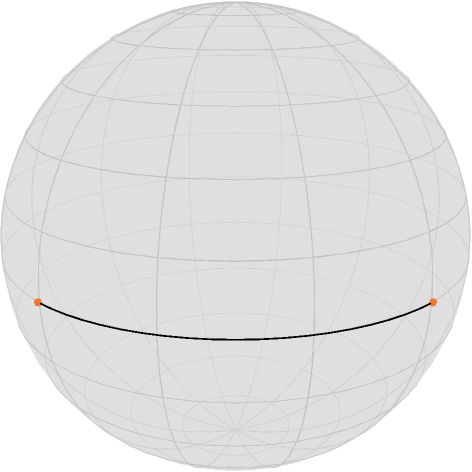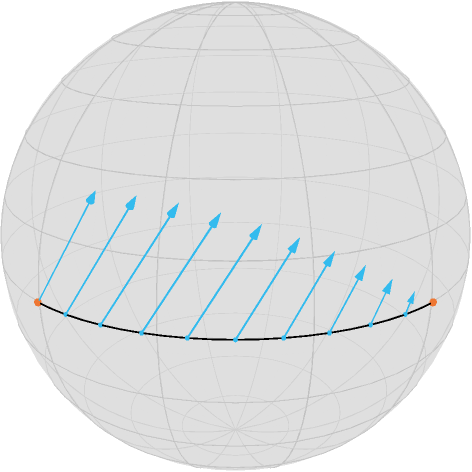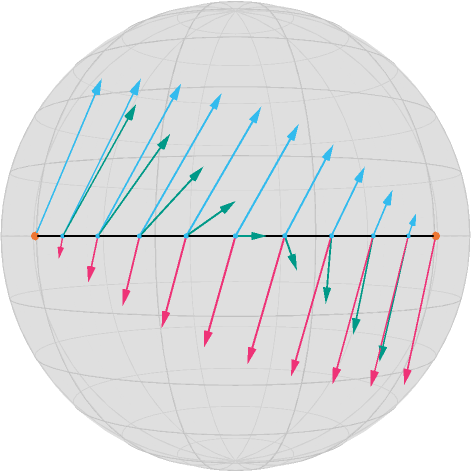# Illustration of Jacobi Fields

This tutorial illustrates the usage of Jacobi Fields within Manopt.jl. For this tutorial you should be familiar with the basic terminology on a manifold like the exponential and logarithmic map as well as shortest geodesics.

We first initialize the packages we need

using Colors, Manopt, Manifolds, PlutoUI

and we define some colors from Paul Tol

begin
black = RGBA{Float64}(colorant"#000000")
TolVibrantMagenta = RGBA{Float64}(colorant"#EE3377")
TolVibrantOrange = RGBA{Float64}(colorant"#EE7733")
TolVibrantCyan = RGBA{Float64}(colorant"#33BBEE")
TolVibrantTeal = RGBA{Float64}(colorant"#009988")
end;

And setup our graphics paths

begin
localpath = join(splitpath(@__FILE__)[1:(end - 1)], "/") # files folder
image_prefix = localpath * "/jacobi_fields"
@info image_prefix
render_asy = false # on CI or when you do not have asymptote, this should be false
end;

Assume we have two points on the equator of the Sphere $\mathcal M = \mathbb S^2$

M = Sphere(2)
Sphere(2, ℝ)
p, q = [[1.0, 0.0, 0.0], [0.0, 1.0, 0.0]]
2-element Vector{Vector{Float64}}:
[1.0, 0.0, 0.0]
[0.0, 1.0, 0.0]

their connecting shortest geodesic (sampled at 100 points)

geodesic_curve = shortest_geodesic(M, p, q, [0:0.1:1.0...]);

Is just a curve along the equator

render_asy && asymptote_export_S2_signals(
image_prefix * "/jacobi_geodesic.asy";
curves=[geodesic_curve],
points=[[p, q]],
colors=Dict(:curves => [black], :points => [TolVibrantOrange]),
dot_size=3.5,
line_width=0.75,
camera_position=(1.0, 1.0, 0.5),
);
render_asy && render_asymptote(image_prefix * "/jacobi_geodesic.asy"; render=2);
PlutoUI.LocalResource(image_prefix * "/jacobi_geodesic.png")where $p$ is on the left and $q$ on the right. We know solve the following task:

Given a direction $X ∈ T_p\mathcal M$, for example

X = [0.0, 0.4, 0.5]
3-element Vector{Float64}:
0.0
0.4
0.5

we move the start point $x$ into, how does any point on the geodesic move?

Or mathematically: Compute $D_p g(t; p,q)$ for some fixed $t∈[0,1]$ and a given direction $X_p$. Of course two cases are quite easy: For $t=0$ we are in $x$ and how $x$ “moves” is already known, so $D_x g(0;p,q) = X$. On the other side, for $t=1$, $g(1; p,q) = q$ which is fixed, so $D_p g(1; p,q)$ is the zero tangent vector (in $T_q\mathcal M$).

For all other cases we employ a jacobi_field, which is a (tangent) vector field along the shortest geodesic given as follows: The geodesic variation $\Gamma_{g,X}(s,t)$ is defined for some $\varepsilon > 0$ as

$$\Gamma_{g,X}(s,t):=\exp{\gamma_{p,X}(s)}[t\log_{g(s;p,X)}p],\qquad s∈(-\varepsilon,\varepsilon),\ t∈[0,1].$$

Intuitively we make a small step $s$ into direction $ξ$ using the geodesic $g(⋅; p,X)$ and from $r=g(s; p,X)$ we follow (in $t$) the geodesic $g(⋅; r,q)$. The corresponding Jacobi field $J_{g,X}$ along $g(⋅; p,q)$ is given by

$$J_{g,X}(t):=\frac{D}{\partial s}\Gamma_{g,X}(s,t)\Bigl\rvert_{s=0}$$

which is an ODE and we know the boundary conditions $J_{g,X}(0)=X$ and $J_{g,X}(t) = 0$. In symmetric spaces we can compute the solution, since the system of ODEs decouples, see for example [doCarmo1992], Chapter 4.2. Within Manopt.jl this is implemented as jacobi_field(M,p,q,t,X[,β]), where the optional parameter (function) $β$ specifies, which Jacobi field we want to evaluate and the one used here is the default.

We can hence evaluate that on the points on the geodesic at

T = [0:0.1:1.0...];

namely

r = shortest_geodesic(M, p, q, T);

The tangent vector now moves as a differential along the geodesic as

W = jacobi_field.(Ref(M), Ref(p), Ref(q), T, Ref(X));

Which can also be called using differential_geodesic_startpoint. We can add to the image above by creating extended tangent vectors the include their base points

V = [Tuple([a, b]) for (a, b) in zip(r, W)];

to add the vectors as one further set to the Asymptote export.

render_asy && asymptote_export_S2_signals(
image_prefix * "/jacobi_geodesic_diff_start.asy";
curves=[geodesic_curve],
points=[[p, q], r],
tangent_vectors=[V],
colors=Dict(
:curves => [black],
:points => [TolVibrantOrange, TolVibrantCyan],
:tvectors => [TolVibrantCyan],
),
dot_sizes=[3.5, 2.0],
line_width=0.75,
camera_position=(1.0, 1.0, 0.5),
);
render_asy && render_asymptote(image_prefix * "/jacobi_geodesic_diff_start.asy"; render=2);
PlutoUI.LocalResource(image_prefix * "/jacobi_geodesic_diff_start.png")The interpretation is as follows: If an infinitesimal change of the start point in direction $X$ would happen, the infinitesimal change of a point along the line would change as indicated.

Note that each new vector is a tangent vector to its point (up to a small numerical tolerance), so the blue vectors are not just “shifted and scaled versions” of $X$.

all([is_vector(M, a, a; atol=1e-15) for a in V])
true

If we further move the end point, too, we can derive that Differential in direction

begin
Y = [0.2, 0.0, -0.5]
W2 = differential_geodesic_endpoint.(Ref(M), Ref(p), Ref(q), T, Ref(Y))
V2 = [Tuple([a, b]) for (a, b) in zip(r, W2)]
end;

and we can combine both vector fields we obtained

V3 = [Tuple([a, b]) for (a, b) in zip(r, W2 + W)];
render_asy && asymptote_export_S2_signals(
image_prefix * "/jacobi_geodesic_complete.asy";
curves=[geodesic_curve],
points=[[p, q], r],
tangent_vectors=[V, V2, V3],
colors=Dict(
:curves => [black],
:points => [TolVibrantOrange, TolVibrantCyan],
:tvectors => [TolVibrantCyan, TolVibrantMagenta, TolVibrantTeal],
),
dot_sizes=[3.5, 2.0],
line_width=0.75,
camera_position=(1.0, 1.0, 0.0),
);
render_asy && render_asymptote(image_prefix * "/jacobi_geodesic_complete.asy"; render=2);
PlutoUI.LocalResource(image_prefix * "/jacobi_geodesic_complete.png")Here the first vector field is still in blue, the second is in magenta, and their combined effect is in teal. Sure as a differential this does not make much sense, maybe as an infinitesimal movement of both start and end point cmobined.

## Literature

doCarmo1992

do Carmo, Manfredo Riemannian Geometry, Birkhäuser Boston, 1992, ISBN: 0-8176-3490-8.